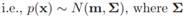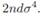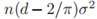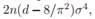# Assume that a large number n of d-dimensional samples has been chosen from a multidimensional Gaussian,  is an arbitrary positive definite covariance matrix. (a) Prove that the distribution of the criterion function Je(1) given in Eq. 82 is normal with mean ndσ2. Express σ in terms of Σ. (b) Prove that the variance of this distribution is (c) Consider a suboptimal partition of the Gaussian by a hyperplane through the sample mean. Show that for large n, the sum of squared error for this partiction is approximately normal with mean  and variance  where σ is given in part (a).

Question
1 views

Assume that a large number n of d-dimensional samples has been chosen from a multidimensional Gaussian,is an arbitrary positive definite covariance matrix.

(a) Prove that the distribution of the criterion function Je(1) given in Eq. 82 is normal with mean ndσ2. Express σ in terms of Σ.

(b) Prove that the variance of this distribution is(c) Consider a suboptimal partition of the Gaussian by a hyperplane through the sample mean. Show that for large n, the sum of squared error for this partiction is approximately normal with meanand variancewhere σ is given in part (a).

### This question hasn't been answered yet.